# 最优模型选择准则：AIC和BIC

• 复杂度惩罚：对应“参数数量”、“训练数据量”，数值变大表明模型复杂度增加，容易过拟合
• 精度惩罚：对应“负log-似然函数”，数值变大表明似然函数降低，模型对数据集的描述能力下降

1、实现参数的稀疏有什么好处？

2、参数值越小代表模型越简单吗？

## AIC

$$AIC=2k-2\ln(L)$$

• k是参数的数量
• L是似然函数

$$AIC=2k+n\ln(RSS/n)$$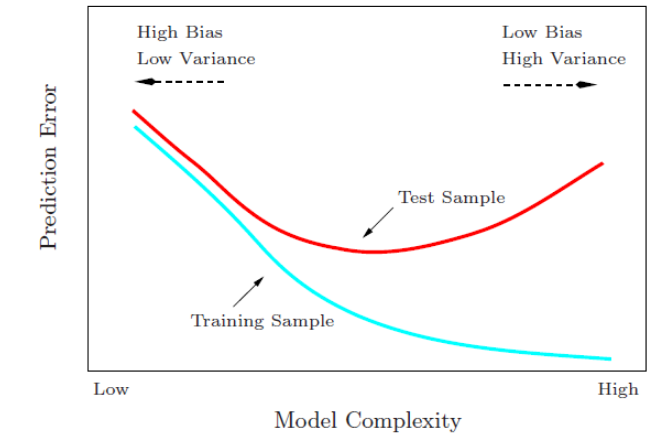## BIC

$$BIC = \ln(n)k – 2ln(L)$$

• k为模型参数个数
• n为样本数量
• L为似然函数

$\ln(n)k$惩罚项在维数过大且训练样本数据相对较少的情况下，可以有效避免出现维度灾难现象。

## AIC和BIC该如何选择？

AIC和BIC的原理是不同的，AIC是从预测角度，选择一个好的模型用来预测，BIC是从拟合角度，选择一个对现有数据拟合最好的模型，从贝叶斯因子的解释来讲，就是边际似然最大的那个模型。

• 构造这些统计量所遵循的统计思想是一致的，就是在考虑拟合残差的同事，依自变量个数施加“惩罚”。

• BIC的惩罚项比AIC大，考虑了样本个数，样本数量多，可以防止模型精度过高造成的模型复杂度过高。
• AIC和BIC前半部分是一样的，BIC考虑了样本数量，样本数量过多时，可有效防止模型精度过高造成的模型复杂度过高。

AIC和BIC前半部分是惩罚项，当n≥8n≥8时，kln(n)≥2kkln(n)≥2k，所以，BIC相比AIC在大数据量时对模型参数惩罚得更多，导致BIC更倾向于选择参数少的简单模型。

## AIC/BIC实战：Lasso模型选择

Lars仅为路径中的每个拐点计算路径解决方案。因此，当只有很少的弯折时，也就是很少的特征或样本时，它是非常有效的。此外，它能够计算完整的路径，而不需要设置任何元参数。与之相反，坐标下降算法计算预先指定的网格上的路径点(本示例中我们使用缺省值)。因此，如果网格点的数量小于路径中的拐点的数量，则效率更高。如果特征数量非常大，并且有足够的样本来选择大量特征，那么这种策略就非常有趣。在数值误差方面，Lars会因变量间的高相关度而积累更多的误差，而坐标下降算法只会采样网格上路径。

import time

import numpy as np
import matplotlib.pyplot as plt

from sklearn.linear_model import LassoCV, LassoLarsCV, LassoLarsIC
from sklearn import datasets

# 这样做是为了避免在np.log10时除零
EPSILON = 1e-4

X = diabetes.data
y = diabetes.target

rng = np.random.RandomState(42)
X = np.c_[X, rng.randn(X.shape, 14)]  # 添加一些不好的特征

# 将最小角度回归得到的数据标准化，以便进行比较
X /= np.sqrt(np.sum(X ** 2, axis=0))

# LassoLarsIC: 用BIC/AIC判据进行最小角度回归

model_bic = LassoLarsIC(criterion='bic')
t1 = time.time()
model_bic.fit(X, y)
t_bic = time.time() - t1
alpha_bic_ = model_bic.alpha_

model_aic = LassoLarsIC(criterion='aic')
model_aic.fit(X, y)
alpha_aic_ = model_aic.alpha_

def plot_ic_criterion(model, name, color):
alpha_ = model.alpha_ + EPSILON
alphas_ = model.alphas_ + EPSILON
criterion_ = model.criterion_
plt.plot(-np.log10(alphas_), criterion_, '--', color=color,
linewidth=3, label='%s 判据' % name)
plt.axvline(-np.log10(alpha_), color=color, linewidth=3,
label='alpha: %s 估计' % name)
plt.xlabel('-log(alpha)')
plt.ylabel('判据')

plt.figure()
plot_ic_criterion(model_aic, 'AIC', 'b')
plot_ic_criterion(model_bic, 'BIC', 'r')
plt.legend()
plt.title('模型选择的信息判据 (训练时间:%.3fs)' % t_bic)
plt.show()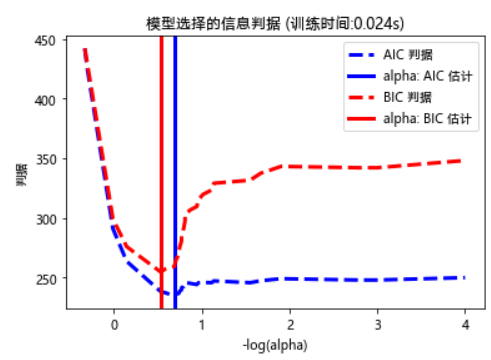import time

import numpy as np
import matplotlib.pyplot as plt

from sklearn.linear_model import LassoCV, LassoLarsCV, LassoLarsIC
from sklearn import datasets

# 这样做是为了避免在np.log10时除零
EPSILON = 1e-4

X = diabetes.data
y = diabetes.target

rng = np.random.RandomState(42)
X = np.c_[X, rng.randn(X.shape, 14)]  # 添加一些不好的特征

# 将最小角度回归得到的数据标准化，以便进行比较
X /= np.sqrt(np.sum(X ** 2, axis=0))

# LassoLarsIC: 用BIC/AIC判据进行最小角度回归

model_bic = LassoLarsIC(criterion='bic')
t1 = time.time()
model_bic.fit(X, y)
t_bic = time.time() - t1
alpha_bic_ = model_bic.alpha_

model_aic = LassoLarsIC(criterion='aic')
model_aic.fit(X, y)
alpha_aic_ = model_aic.alpha_

# LassoCV: 坐标下降

# 计算路径
t1 = time.time()
model = LassoCV(cv=20).fit(X, y)
t_lasso_cv = time.time() - t1

# 显示结果
m_log_alphas = -np.log10(model.alphas_ + EPSILON)

plt.figure()
ymin, ymax = 2300, 3800
plt.plot(m_log_alphas, model.mse_path_, ':')
plt.plot(m_log_alphas, model.mse_path_.mean(axis=-1), 'k',
label='平均', linewidth=2)
plt.axvline(-np.log10(model.alpha_ + EPSILON), linestyle='--', color='k',
label='alpha:CV估计')

plt.legend()

plt.xlabel('-log(alpha)')
plt.ylabel('均方惨差')
plt.title('每折上的均方残差: 坐标下降法'
'(训练时间: %.2fs)' % t_lasso_cv)
plt.axis('tight')
plt.ylim(ymin, ymax)
plt.show()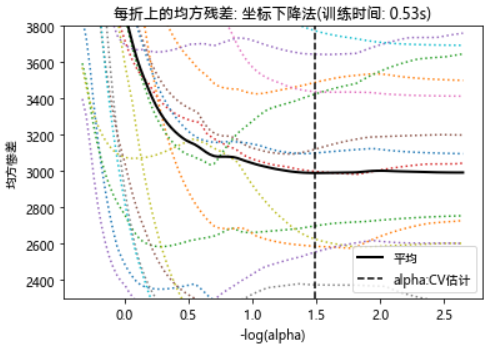import time

import numpy as np
import matplotlib.pyplot as plt

from sklearn.linear_model import LassoCV, LassoLarsCV, LassoLarsIC
from sklearn import datasets

# 这样做是为了避免在np.log10时除零
EPSILON = 1e-4

X = diabetes.data
y = diabetes.target

rng = np.random.RandomState(42)
X = np.c_[X, rng.randn(X.shape, 14)]  # 添加一些不好的特征

# 将最小角度回归得到的数据标准化，以便进行比较
X /= np.sqrt(np.sum(X ** 2, axis=0))

# LassoLarsIC: 用BIC/AIC判据进行最小角度回归

model_bic = LassoLarsIC(criterion='bic')
t1 = time.time()
model_bic.fit(X, y)
t_bic = time.time() - t1
alpha_bic_ = model_bic.alpha_

model_aic = LassoLarsIC(criterion='aic')
model_aic.fit(X, y)
alpha_aic_ = model_aic.alpha_

ymin, ymax = 2300, 3800

# LassoLarsCV: 最小角度回归法

# Compute paths
print("Computing regularization path using the Lars lasso...")
t1 = time.time()
model = LassoLarsCV(cv=20).fit(X, y)
t_lasso_lars_cv = time.time() - t1

# Display results
m_log_alphas = -np.log10(model.cv_alphas_ + EPSILON)

plt.figure()
plt.plot(m_log_alphas, model.mse_path_, ':')
plt.plot(m_log_alphas, model.mse_path_.mean(axis=-1), 'k',
label='平均', linewidth=2)
plt.axvline(-np.log10(model.alpha_), linestyle='--', color='k',
label='alpha CV')
plt.legend()

plt.xlabel('-log(alpha)')
plt.ylabel('均方惨差')
plt.title('每折上的均方残差: 最小角度回归法'
'(训练时间: %.2fs)' % t_lasso_lars_cv)
plt.axis('tight')
plt.ylim(ymin, ymax)

plt.show()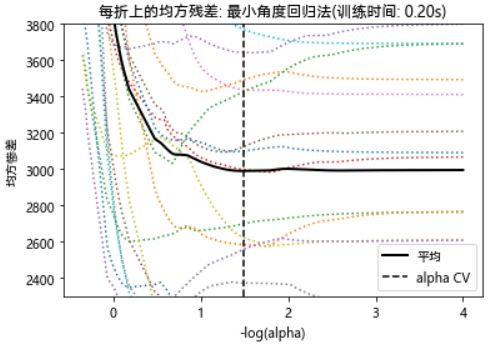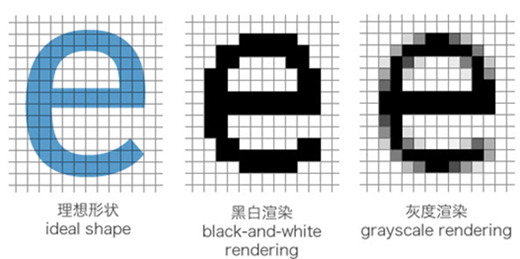##### 网站与APP开发中的字体设置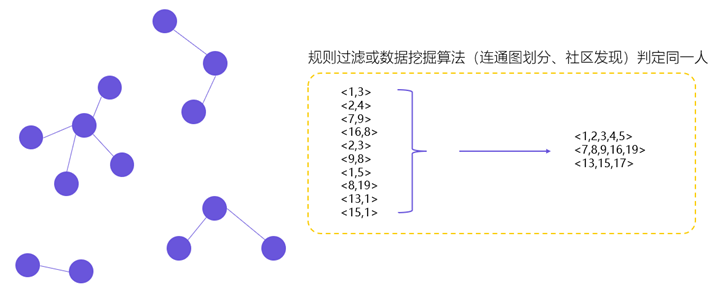##### 用户体系搭建之ID-Mapping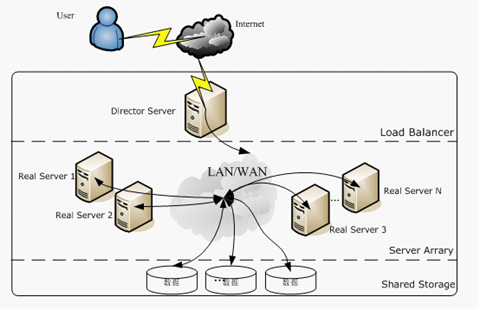## 2 Replies to “最优模型选择准则：AIC和BIC”

1. Ashley说道：

讲的很清楚，还有实例，感谢

2. chep说道：

aic, bic 准则 lr模型，决策树模型 怎么用呢？